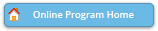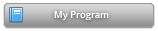#### Abstract Details

 Activity Number: 20 Type: Topic Contributed Date/Time: Sunday, July 31, 2016 : 2:00 PM to 3:50 PM Sponsor: Section on Statistical Learning and Data Science Abstract #320195 View Presentation Title: Viewing a Permutation as a Measure on the Unit Square Author(s): Sumit Mukherjee* Companies: Columbia University Keywords: Permutation ; Measure ; Copula ; Mallows model ; Pseudolikelihood Abstract: The idea of viewing a permutation as a unit square first originated in Combinatorics, and is motivated by dense graph theory. As application of this representation, we are able to compute limiting properties of various statistics on permutations for various non uniform probability models of interest. Examples include the number of fixed points, number of cycles of given length and number of inversions. We are also able to analyze behavior of the degree sequence of random permutation graphs, which are of interest in Discrete Mathematics and Computer Science. Focusing on Statistics, we can analyze a wide class of non uniform probability measures on permutations which include Mallows model with Spearman's correlation as a special case. As a result of this analysis, we compute the limiting log normalizing constant for such models, and give an iterative algorithm for computing this limit. We also show consistency of Maximum Likelihood Estimate and Pseudo-likelihood in these models.

Authors who are presenting talks have a * after their name.